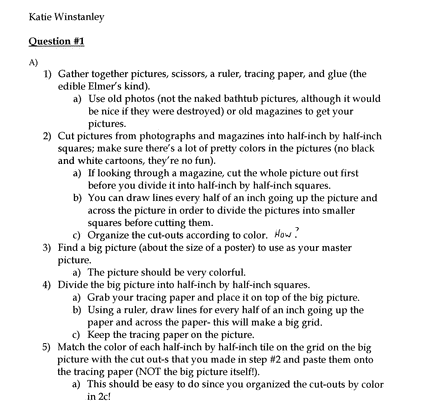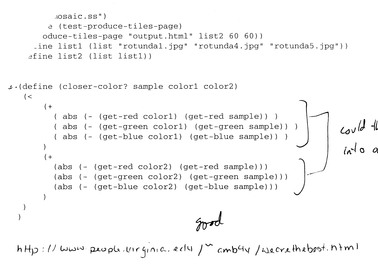University of Virginia, Department of Computer Science CS200: Computer Science, Spring 2002

 Problem Set 1: Mosaics - Selected Answers

 Honor Code Reminder: If you are currently taking CS200, it is a violation of the course pledge to look at Problem Set answers and discussions from previous years. Please don't do it.

 Question 1: Consider the problem of making a photomosaic without using a computer. Describe two different ways of dividing that problem into subproblems. Continue dividing those sub-problems until you have steps simple enough that a none-too-brilliant kindergartner could follow them to create a photomosaic.### Scheme Expressions

 Question 2, 3: For each fragment below, either: Explain why the fragment is not a valid Scheme expression; or, Predict what value the expression will evaluate to.
1. 200
Evaluation Rule 1: Primitives. If the expression is a primitive, it is self-evaluating.

200

2. (+ 100 100)
Evaluation Rule 3: Application. If the expression is an application:
(a) Evaluate all the subexpressions of the combination (in any order)
(b) Apply the value of the first subexpression to the values of all the other subexpressions.

Subexpression + evaluates to + (by Evaluation Rule 1: Primitives.)
Subexpression 100 evaluates to 100 (by Evaluation Rule 1: Primitives.)
Subexpression 100 evaluates to 100 (by Evaluation Rule 1: Primitives.)

Application Rule 1: Primitives. If the procedure to apply is a primitive, just do it.

So (+ 100 100) evaluates to 200

3. +
Evaluation Rule 1: Primitives. If the expression is a primitive, it is self-evaluating.

+
DrScheme displays this as <primitive:+>

4. (100 + 100)
Evaluation Rule 3: Application. If the expression is an application:
(a) Evaluate all the subexpressions of the combination (in any order)
(b) Apply the value of the first subexpression to the values of all the other subexpressions.

Subexpression 100 evaluates to 100 (by Evaluation Rule 1: Primitives.)
Subexpression + evaluates to + (by Evaluation Rule 1: Primitives.)
Subexpression 100 evaluates to 100 (by Evaluation Rule 1: Primitives.)

The first subexpression evaluates to 100, but there is no application rule to apply a non-procedure, so this cannot be evaluated. DrScheme produces this error:
procedure application: expected procedure, given: 100; arguments were: #<primitive:+> 100

5. (> 200 101)
Evaluation Rule 3: Application. If the expression is an application:
(a) Evaluate all the subexpressions of the combination (in any order)
(b) Apply the value of the first subexpression to the values of all the other subexpressions.

Subexpression > evaluates to > (by Evaluation Rule 1: Primitives.)
Subexpression 200 evaluates to 200 (by Evaluation Rule 1: Primitives.)
Subexpression 101 evaluates to 101 (by Evaluation Rule 1: Primitives.)

Application Rule 1: Primitives. If the procedure to apply is a primitive, just do it.

So (> 200 101) evaluates to #t

6. (and (> 200 101) (> 200 588))
7. (if (> 200 101) "good move" "try again")
8. (if (not "cookies") "eat" "starve")
We won't show all the rules for these, but you should be able to work through them to get the same results as the interpreter.

Note the the quotation marks are important. With them "eat" is a primitive that self-evaluates to "eat"; without them eat is a name that is evaluated according to Evaluation Rule 2. Since there is no value assocuated with the name eat, it cannot be evaluated and would produce an error.

 Question 4: Define a Scheme function that tests produce-tiles-page. Your test function should produce a .html file that displays some images. The file images.zip contains some images you can use once you have unziped this file. Look at the .html file you produce both in a web browser and in a text editor. definitions file.

We need to create a list of lists of images. One way (done by Jacques Fournier and Victor Clay Yount) is:

```(define (test-tiles-page)
(produce-tiles-page
"test.html"
(list
(list "rotunda1.jpg" "rotunda4.jpg")
(list "rotunda1.jpg" "rotunda4.jpg"))
200 200))
```
Another way is to use the directory-list function like Spencer Stockdale and Nate Parks did:
```(define thelist (list (directory-list)))
(define (test-produce-tiles-page)
(produce-tiles-page "test.html" thelist 50 50))
```
Note the use ot list before (directory-list) to turn it into a list of lists.

 Question 5: Try calling generate-sample-points in the interaction window with different parameters. Are the results what you expect? Print out your interactions buffer with notes on any surprising results.

There are several problems with generate-sample-points:

1. It starts at (0, 0), so the samples will be slanted towards the upper left corner of the images. It would be better (but a bit more complicated) if the samples we're spread out evenly over the tile.
2. The number of points returned does not match exactly the number requested. This is because it calculates the sample spacing using (/ width (sqrt num-points)), and then scales the height spacing. If the region to be sampled is not a square, the wrong number of points is sampled. The other reason the number of points is different is because of rounding - we sample exact pixels, but the sample spacing may be a non-integer.
 Question 6: Write a function that can be passed as color-comparator. Define your function in the definitions file you created for Question 4. Use your function to create a photomosaic web page.

There are many good ways to compare colors. Here are a few examples:

Russel Patrick O'Reagan and Andrew Hanlin's answer:

```(define (square x) (* x x))

(define (compare-color
sample
color1
color2)
(<
(+
(square (- (get-red color1) (get-red sample)))
(square (- (get-blue color1) (get-blue sample)))
(square (- (get-green color1) (get-green sample))))
(+
(square (- (get-red color2) (get-red sample)))
(square (- (get-blue color2) (get-blue sample)))
(square (- (get-green color2) (get-green sample))))
)
)
```
Output